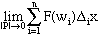Subject: Calculus Proof Mark Spilker college student I have a proof that I cannot do here it goes. Let F(x)= 1 if x is a rational number 0 if x is an irrational number Prove the function is not intregrable on the interval (0,1). Hint: Show that no matter how small the norm of the partition, the Riemann Sum for the SigmaNotation F(wi) DeltaiX is not unique. I look forward to hearing back from you Hi Mark, To prove the integrability of F(x) from 0 to 1 you need to consider the limitwhere |P| is the norm of the partition and wi is a point in the interval from xi-1 to xi. This limit must exist and its value must NOT depend on the choice of wi. With the function that you have, no matter how small |P|, you can always find a rational and an irrational in each interval from xi-1 to xi. If you always choose wi to be rational then the limit is 1, and if you always choose wi to be irrational then the limit is 0. Thus the value of the limit depends on the choice of wi and hence the integral does not exist. I hope this helps, Harley Go to Math Central